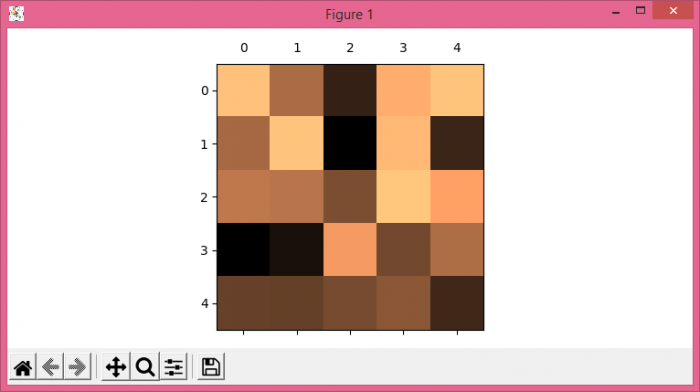# How to move labels from bottom to top without adding "ticks" in Matplotlib?

To move labels from bottom to top without adding ticks, we can take the following steps −

• Set the figure size and adjust the padding between and around the subplots.
• Create random data of 5☓5 dimension matrix.
• Display the data as an image, i.e., on a 2D regular raster using imshow() method.
• Use tick_params() method to move labels from bottom to top.
• To display the figure, use show() method.

## Example

import numpy as np
from matplotlib import pyplot as plt

plt.rcParams["figure.figsize"] = [7.50, 3.50]
plt.rcParams["figure.autolayout"] = True

data = np.random.rand(5, 5)

plt.imshow(data, cmap="copper")
plt.tick_params(axis='both', which='major',
labelsize=10, labelbottom=False,
bottom=True, top=False, labeltop=True)

plt.show()

## Output